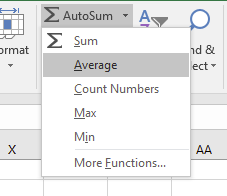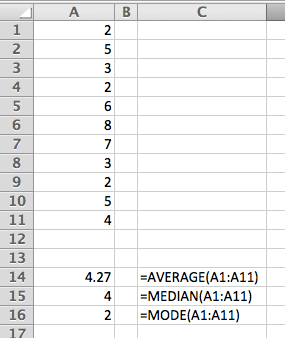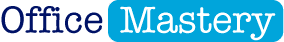# 3 ways to calculate an AVERAGE in Excelby Jason Morrell
March 9, 2015There are many situations where it’s useful to calculate an average. For example,

• What is the average height of pupils in a class?
• What is the average monthly electricity bill over the course of a year?
• What is the average amount of sick days taken by employees? By Department? By Men? By Women?

Of course there are situations where it is not useful. For example (and I don’t mean to be rude)

On average humans have one boob and one testicle !

And don’t you think it’s odd that

80% of drivers consider themselves better than average!

How does that work? We can’t all be right, can we!

Here's what is covered in this post.

## 1.  The 3 ways to calculate an average value

You may remember from your maths lessons at school that there are 3 types of average: MEDIAN, MODE and MEAN.

• To work out the MEDIAN, Excel sorts your data values into order (behind the scenes), then selects the middle value.
• To work out the MODE, Excel sorts your data values into order (behind the scenes), then selects the value that occurs the most frequently.
• To calculate the MEAN, Excel totals all the data values (behind the scenes), then divides the answer by the number of data values in the list.

## 2. How to calculate a MEAN AVERAGE in Excel

The simplest and most common way to calculate the average value for a set of numbers is to use Excel’s AVERAGE function. This function uses the MEAN method which totals the data then divides the answer by the number of values.

The structure of the AVERAGE function is

For example:

or

###### =AVERAGE(A1:A11)

This function totals all items within the brackets then divides by the number of items. It can be selected from the AutoSum drop down menu, or by typing it directly into the cell.

If you have values in cells A1 to A11.

To use the AutoSum tool to calculate the average:

1.  Select the 11 cells.

2.  Click the drop-down arrow on the AutoSum icon.

3.  Choose AVERAGE from the list.Figure 01: There are 5 basic functions available on the AutoSum dropdown menu

Or if you want to write the formula manually:

1.  Select a blank cell that will contain the calculated average.

2.  Type =AVERAGE(
(You cannot use AVG or any other shortened version of AVERAGE).

3.  With your mouse, select cells A1 to A11.

4.  Type )    (the closing bracket).

5.  Press Enter.

Using either technique you should end up with

## 3. How to calculate the MEDIAN value in Excel

The second method is to use Excel’s MEDIAN function. This function looks at the sorted sequence of data (behind the scenes) then selects the middle value.

The structure of the MEDIAN function is

For example:

## 4. How to calculate the MODE value in Excel

The third and final method is to use Excel’s MODE function. This function looks at the sorted sequence of data (behind the scenes), then selects the most common value.

Think of the ‘MO’. The MOde function selects the MOst common value.

The structure of the MODE function is

For example:

## 5. Summary

Pulling everything together, here’s how the 3 different functions compare:Figure 02: Three different types of average in Excel

## 6. What next?

Please feel free to post any questions you have into the comments below. And before you leave this page, I want to give you one quick little action item!

Enjoy the rest of your day!Jason Morrell

Jason loves to simplify the hard stuff, cut the fluff and share what actually works. Things that make a difference. Things that slash hours from your daily work tasks. He runs a software training business in Queensland, Australia, lives on the Gold Coast with his wife and 4 kids and often talks about himself in the third person!

SHARE

more from## Excel Sorting Secrets Revealed: Sort Like a Pro

Excel Sorting Secrets Revealed: Sort Like a Pro

## Using Images to Add Visual Interest and a Professional Finish to Any Spreadsheet

Using Images to Add Visual Interest and a Professional Finish to Any Spreadsheet

JOIN THE DISCUSSION